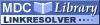Search
Browse
Statistics
Feeds

# The use of small-angle scattering and the maximum-entropy method for shape-model determination from distance-distribution functionsPDF - Requires a PDF viewer such as GSview, Xpdf or Adobe Acrobat Reader 859kBItem Type: Article The use of small-angle scattering and the maximum-entropy method for shape-model determination from distance-distribution functions Mueller, J.J. and Hansen, S. and Puerschel, H.V. The maximum-entropy method is well established for the analysis of scattering data [Bricogne (1993). Acta Cryst. D49, 37-60]. For this method, prior structure knowledge can be included in the structure determination. This prior estimate is an essential element for a successful application of the maximum-entropy method. The most likely prior estimate can be found by maximization of the entropy. With the assumption a priori of a special type of structure model, the unknown parameters can be calculated from real-space functions. For practical use, analytical expressions for the Fourier transform of model scattering curves, the distance-distribution function of the models, are of interest. Formulas are presented for rotational ellipsoids, Gaussian chains and two-phase spheres, and a parameter estimation by the program MAXENT is demonstrated for the ellipsoidal shape of cytochrome c using theoretical X-ray scattering curves calculated from atomic coordinates. The calculated dimensions of prolate and oblate ellipsoids agree within the error limits with the direct structure-related inertia-equivalent ellipsoid of the molecule. Furthermore, error limits have been determined from the a posteriori probability or 'evidence' function for the model parameters. To avoid overinterpretation of the scattering data, the real number of degrees of freedom is calculated for noisy data. This measure of information content is almost independent of the collimation distortion but strongly influenced by the statistical noise in the scattering data. The numerical value is smaller than the ideal number of degrees of freedom provided by the information theory. X-Ray-Scattering Journal of Applied Crystallography 0021-8898 International Union of Crystallography 29 Pt 5 547-554 1 October 1996 https://doi.org/10.1107/S0021889896004840

Repository Staff Only: item control page